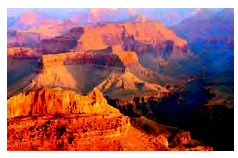Chapter 9, Problem 11REMathematical Excursions (MindTap C...

4th Edition
Richard N. Aufmann + 3 others
ISBN: 9781305965584

Solutions

Chapter
SectionMathematical Excursions (MindTap C...

4th Edition
Richard N. Aufmann + 3 others
ISBN: 9781305965584
Textbook Problem

Meteorology In June, the temperature at various elevations of the Grand Canyon can be approximated by the equation T = − 0.005 x + 113.25 , where T is the temperature in degrees Fahrenheit and x is the elevation (distance above sea level) in feet. Use this equation to find the elevation at Inner Gorge, the bottom of the canyon, where the temperature is 101 ° F .The Grand Canyon

To determine

The elevation at Inner Gorge when the temperature is 101 F.

Explanation

Given information:

The temperature at various elevations of the Grand Canyon is approximated by the equation T=0.005x+113.25. The temperature at Inner Gorge is 101 F.

Calculation:

T=0.005x+113.25(Given)

The temperature T is 101 F.

Therefore, the given equation becomes 101=0.005x+113.25

By subtraction property, subtract both sides by 113.25

Still sussing out bartleby?

Check out a sample textbook solution.

See a sample solution

The Solution to Your Study Problems

Bartleby provides explanations to thousands of textbook problems written by our experts, many with advanced degrees!

Get Started

In Problems 76-82, perform the indicated operations and simplify. x1x2x2xx22x3+1x2

Mathematical Applications for the Management, Life, and Social Sciences

Solve the equations in Exercises 126. 1x211+1=0

Finite Mathematics and Applied Calculus (MindTap Course List)

Evaluate the integral, if it exists. x31+x4dx

Single Variable Calculus: Early Transcendentals, Volume I

Evaluate the integral. 25. 3x3x2+6x4(x2+1)(x2+2)dx

Single Variable Calculus: Early Transcendentals